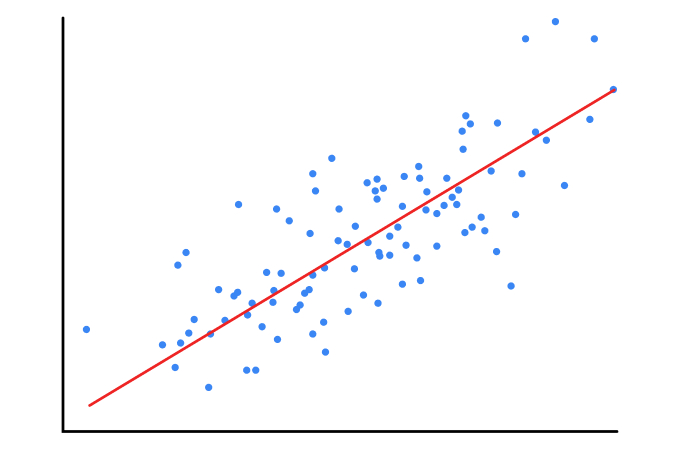### Subscribe Us# Linear Regression(With Gradient Descent ) Fully Explained| Machine Learning

## WHAT IS LINEAR REGRESSION:

### Suppose you are given a equation: y=2x1+3x2+4x3+1 and you are said to find the value at any point (1,1,1) corresponds to x1, x2, x3 respectively.You'll simply put the value of x1, x2, x3 into equation and tell me the answer :10,Right?But What if you are given different set of (x1, x2, x3,y) and you are said to find the equation.Here's what,Linear Regression Comes into picture.It helps us to find out or fit a Linear equation to datasets  given.Above equation can be easily transformed to following form.If we suppose:
θ=[2,3,4]
X=[x1, x2, x3]
c=1
Above equation can be written as : Y=θ.X+C
Linear Regression help us to find the value of θ and c
For this post we will be limited to a single line equation, multidimensional linear equation can also be done in similar way.
X      Y
2      7
3    10
4    13
5    16
After Seeing above data set, we can clearly say ,there is a relation ,
Y=2*X +1
is there.But how will we get above equation using linear regression , will try to understand in this post.
•  Assume a line yp=mx+c
• Cost Function : Our cost function is Measured Squared Error(MSE) i.e Average squared difference of observed value and predicted value.
Cost Function=Mean Squared Error(MSE) = (1/n)Σ(y - yp)2
J(m,c) = (1/n)Σ(y-(mx+c))2

J(m,c) is our cost function now which depends on m and c, as z was depending upon x and y in post of gradient descent.
Now,

Assume,

### Iterations:

(m)new=(m)old η*(∂j/∂m)

### (c)new=(c)old - η*(∂j/∂c)

How many times you want to iterate? It depends upon you or you can fix a condition like cost<=0.000001

### Try Below given Code:

Python Code to implement this